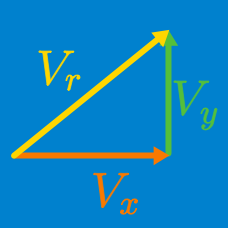Classical Mechanics

Position Vectors

A car at point $A$ on a straight road goes west for $20$ seconds, arriving at point $B$ which is $200$ m away from $A.$ The car then heads back to the east for $30$ seconds, arriving at point $C$ which is $800$ m away from $B.$ What is the displacement of the car from point $A$?

Assume that $+$ is east and $-$ is west.

One morning, you wake up and decide to take a jog through the town. You take $54 \text{ seconds}$ to run $250 \text{ m}$ straight north, then you turn right and take $42 \text{ seconds}$ to run $178 \text{ m}.$ Then you turn right again and run down the street for $9 \text{ seconds}$ covering $30 \text{ m}$ until you to stop. Assuming that the coordinates of your home are the origin $(0, 0),$ find the position vector of the place where you stop.

Take eastward as $+\hat{i}$ and northward as $+\hat{j} .$

A soccer player undergoes two successive displacements: $\Delta \vec{r_A} = (28 \text{ m})\hat{i} + (8\text{ m})\hat{j} \text{ followed by } \Delta \vec{r_B} = (-28\text{ m}) \hat{i}+(7\text{ m}) \hat{j}.$ What is the total displacement of the soccer player?

The motion of a creature in three dimensions can be described by the following equations for positions in $x, y$ and $z$ directions: \begin{aligned} x(t)&=3t^2 + 6 \\ y(t)&=- t^2 + 3t - 2 \\ z(t)&= 3t + 1. \end{aligned} Find the position vector of the creature at $t = 3.$The Andromeda galaxy is a giant spiral cluster of stars whose mass is that of $300$ billion Suns. You can see it with the naked eye as a faint elongated cloud in the night sky. Inasmuch as it subtends an angle of $4.1^{\circ}$ and is known to be larger than our own galaxy [$163 \times 10^3$ light-years (units of ly) in diameter for Andromeda as compared to $100 \times 10^3$ light-years for our galaxy], how far away is it in light-years?

×